# Centroid

(diff) ← Older revision | Latest revision (diff) | Newer revision → (diff)
Jump to: navigation, search

of a set of points in Euclidean space

Considerpoints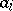,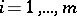, in, with positive weightsattached to them. Then the centroid of this system is the point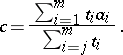This is also the centre of gravity, or barycentre, or centre of mass (in the sense of mechanics) of these mass points. The concept belongs to affine geometry rather than to the vector space (Euclidean space), in the sense that the vector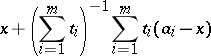is obviously independent of.

If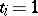,, the term equi-barycentre is sometimes used. The concept can be extended to the case of possibly negative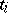(electric charges), see [a1].

A related concept is that of the centre of a compact setwith non-empty interior in, which is defined as:whereis Lebesgue measure. This is the centre of mass, in the sense of mechanics, of the bodywith uniform mass density. Here, a weight function (mass distribution) could be introduced.

For a triangle, the centroid of the three corners, each with weight unity, is the intersection point of the three medians of the triangle (see Plane trigonometry; Median (of a triangle)). This is also the centre of the convex hull of the three corners (Archimedes' theorem). This is no longer necessarily true for four points in the plane.

The centroid ofwith weights,, minimizes the weighted sum of the squared distances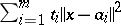.

See also Chebyshev centre of a bounded set.

How to Cite This Entry:
Centroid. Encyclopedia of Mathematics. URL: http://encyclopediaofmath.org/index.php?title=Centroid&oldid=13379
This article was adapted from an original article by M. Hazewinkel (originator), which appeared in Encyclopedia of Mathematics - ISBN 1402006098. See original article